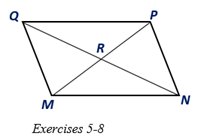Chapter 4.1, Problem 5E### Elementary Geometry for College St...

6th Edition
Daniel C. Alexander + 1 other
ISBN: 9781285195698

#### Solutions

Chapter
Section### Elementary Geometry for College St...

6th Edition
Daniel C. Alexander + 1 other
ISBN: 9781285195698
Textbook Problem
12 views

# For Exercises 5 to 8, MNPQ is a parallelogram with diagonals Q N - and M P - .a) If Q N = 12.8 , find QR. b) If M R = 5.3 , find MP.

To determine

a.

To find:

The length of side find QR in the parallelogram MNPQ.

Explanation

Given:

MNPQ is a parallelogram with diagonals QN- and MP- and QN=12.8.

Corollary:

The diagonals of a parallelogram bisect each other.

Calculation:

In the given parallelogram MNPQ, given that the diagonals QN- and MP- and QN=12.8

To determine

b.

To find:

The length of MP in the parallelogram MNPQ.

### Still sussing out bartleby?

Check out a sample textbook solution.

See a sample solution

#### The Solution to Your Study Problems

Bartleby provides explanations to thousands of textbook problems written by our experts, many with advanced degrees!

Get Started

#### Convert the expressions in Exercises 8596 radical form. 45x3/2

Finite Mathematics and Applied Calculus (MindTap Course List)

#### In Exercises 99106, factor out the greatest common factor from each expression. 102. 3x2/3 2x1/3

Applied Calculus for the Managerial, Life, and Social Sciences: A Brief Approach

#### 0 1 −∞ does not exist

Study Guide for Stewart's Single Variable Calculus: Early Transcendentals, 8th

#### Describe how replication protects against fraud being committed in research.

Research Methods for the Behavioral Sciences (MindTap Course List)